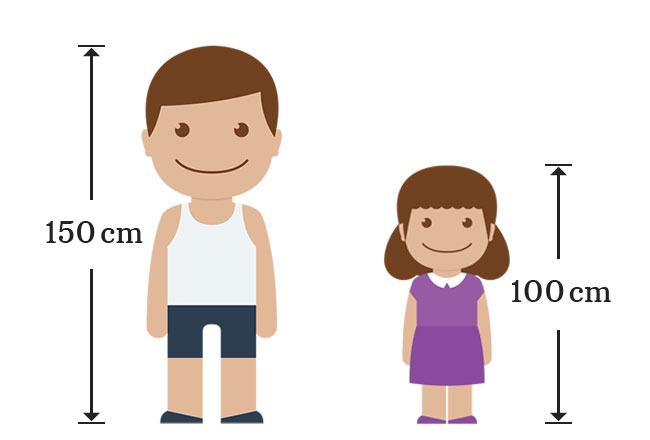# Rational numbers

A number that appears as a ratio of any two integers is called a rational number.

## Introduction

The integers are often appeared in antecedent and consequent positions of the ratio in some cases. The ratio of them is also a number and it is called as a rational number.

### Examples

$\dfrac{1}{4}$, $\dfrac{-7}{2}$, $\dfrac{0}{8}$, $\dfrac{11}{8}$, $\dfrac{15}{5}$, $\dfrac{14}{-7}$, $\cdots$#### Usage

The rational numbers are mainly used to represent the fractions in mathematical form.

#### Rules of formation

There are two rules for forming the rational numbers by the integers.

1. The antecedent can be any integer.
2. The consequent should be a non-zero integer.

#### Note

$10$ and $2$ are two integers and find the ratio of $10$ to $2$ by the division.

$Ratio \,=\, \dfrac{10}{2}$

It is a rational number basically and now, find their quotient.

$\implies$ $\dfrac{10}{2}$ $\,=\,$ $5$

It proves that a rational number can be an integer but an integer may not always be a rational number.

#### Formation

The heights of a boy and his sister are $150 \, cm$ and $100 \, cm$ respectively.Calculate the ratio of boy’s height to his sister’s height.

$Ratio \,=\, \dfrac{150}{100}$
$\implies$ $\require{cancel} Ratio \,=\, \dfrac{\cancel{150}}{\cancel{100}}$
$\implies$ $\require{cancel} Ratio \,=\, \dfrac{3}{2}$

Similarly, calculate the ratio of girl’s height to her brother’s height.

$Ratio \,=\, \dfrac{100}{150}$
$\implies$ $\require{cancel} Ratio \,=\, \dfrac{\cancel{100}}{\cancel{150}}$
$\implies$ $\require{cancel} Ratio \,=\, \dfrac{2}{3}$

$\dfrac{2}{3}$ and $\dfrac{3}{2}$ are two ratios but $2$ and $3$ are integers. So, if any two integers are expressed in ratio form, then they are called the rational numbers. Therefore, $\dfrac{2}{3}$ and $\dfrac{3}{2}$ are called as the rational numbers.

#### Representation

The collection of all rational numbers can be represented as a set and denoted by $Q$, which is a first letter of the “Quotient”. The rational numbers are infinite. So, the set of rational numbers is called as an infinite set.

$Q$ $\,=\,$ $\Big\{\cdots, -2, \dfrac{-9}{7}, -1, \dfrac{-1}{2}, 0, \dfrac{3}{4}, 1, \dfrac{7}{6}, 2, \cdots\Big\}$

Latest Math Topics
Jun 26, 2023
Jun 23, 2023

Latest Math Problems
Jul 01, 2023
Jun 25, 2023
###### Math Questions

The math problems with solutions to learn how to solve a problem.

Learn solutions

Practice now

###### Math Videos

The math videos tutorials with visual graphics to learn every concept.

Watch now

###### Subscribe us

Get the latest math updates from the Math Doubts by subscribing us.